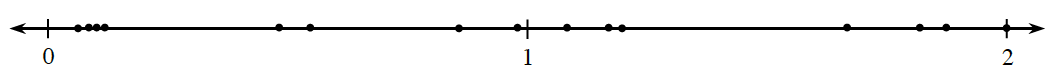### Home > CC1MN > Chapter 5 Unit 5B > Lesson CC1: 5.1.4 > Problem5-36

5-36.

When making estimates, it is sometimes useful to approximate unfamiliar fractions by comparing them to numbers that are more familiar to you. Homework Help ✎

1. Copy the number line below on your paper, including the dots. Keep the dots in the same positions. Label each dot with one of the fractions from the list given below.

$\frac{1}{12},\frac{7}{6},\frac{13}{12},\frac{15}{9},\frac{6}{100},\frac{6}{7},\frac{30}{16},\frac{2}{17},\frac{12}{25},\frac{2}{20},\frac{98}{100},\frac{6}{11},\frac{6}{5},\frac{4}{2},\frac{20}{11}$

It may be helpful to do parts (b) through (e) first before labeling the dots.

2. Which of these fractions are greater than or equal to $1\frac{1}{2}$?

These fractions will have a numerator which is at least $150\%$of the denominator.

$\frac{15}{9},\frac{4}{2},\frac{30}{16},\frac{20}{11}$

3. Which of these fractions are close to the number $1$?

These fractions will have numerators and denominators which are quite close.

4. Which of these fractions are close to $\frac { 1 } { 2 }$?

A fraction fits this description if the numerator is close to half the value of the denominator.

$\frac{12}{25}\text{ and } \frac{6}{11}$

5. Which of these numbers are close to 0?• These fractions have a small numerator and a large denominator.